## Probabilities in Quantum Mechanics and  the Preparation of Dynamical Properties

Exploring the double slit experiment, we find that quantum mechanics makes only statistical predictions about the results of measurements. A typical experiment consists of preparation, interaction, and measurement.The notion of preparation is an important conceptual foundation in the study of quantum mechanics. It is introduced by considering Newton´s prism experiment. The results show in which way the notions of preparation, quantum mechanical state and wave function are interconnected.

 Content: 1. The double slit simulation program 2. Single electrons in the double slit experiment 3. Preparation, interaction, and measurement 4. Preparation and dynamical properties      a) Newton´s prism experiment with light      b) Preparation and test 5. Preparation and quantum mechanical states

### 1. The double-slit simulation program

The simulation program "double slit" forms the basis for this and some of the following lessons. The program provides an interactive lab, where experimental parameters can be modified freely. Their influence on the experiment´s result can then be studied.

Please install the program now by executing the file "Doppelspalt_V31.exe". If you are not in posession of this file, you can download it here.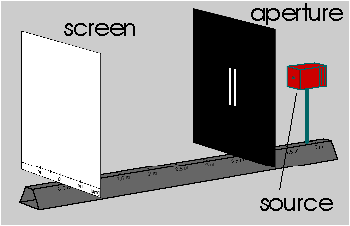The lab consists primarily of three components (see figure):
• a source that emits different types of quantum objects (electrons, photons, atoms) and classical objects. If you click with the mouse on the source, a window appears where you can select the species and adjust the energy of the emitted objects;
• an aperture with a single or double slit. Their width and separation can be varied by clicking;
• a screen where the objects are detected.
When you start the program you will see also a lamp between aperture and screen. It is not needed at the moment and can be ignored.

Now take some time to test and explore the program. You can switch on the source in the control panel on the right side (or simply by clicking on the support rod of the source).

### 2. Single electrons in the double-slit experiment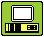Now we perform a simulation experiment with the double-slit program. Choose electrons with an energy of 20 keV, a slit width of 150 nm, and a slit separation of 1000 nm. After switching on the source, electrons are detected on the screen. They lead to point-like spots at apparently random positions. You will notice that the spots form a pattern as more electrons are detected (the process can be accelerated with the "Speed" key). It resembles the well-known double-slit interference pattern of classical optics (figure below).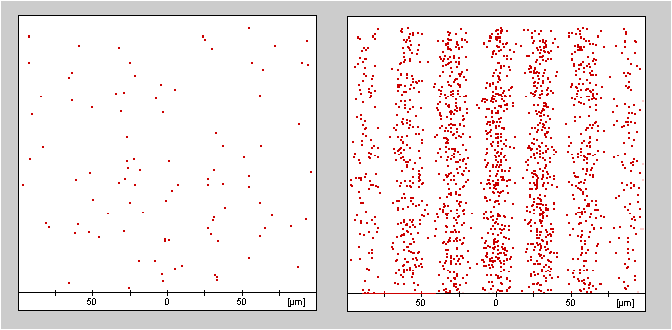Ordinarily, this experiment is used to demonstrate the wave and particle nature of quantum matter. This duality of matter is treated in depth in another lesson. In the following, we will instead be dealing with another question: what can we predict about the position where an electron leaves a spot on the screen?Turn off the source and look at the pattern that the electrons formed on the screen. Suppose you were to send in a single electron through the slits. Can you predict at which position on the screen the electron will be detected?

Perform the experiment by switching on the source for a short time. A new spot appears on the screen and it was not likely that you predicted its exact location.The prediction becomes more successful if we modify the experiment a little. Now, 100 electrons are to be added. You can probably predict with a good accuracy at which places it is likely to find many electrons and at which positions only few are found.

The difference between the two experiments lies in the fact that in the second experiment we changed the rules of the game. Instead of making a prediction about a single event, we made a probability statement. In fact, the success of quantum mechanics has its basis in its statistical predictions. In quantum mechanics, there is no means of predicting the results of single events (except for the trivial cases where the probability is zero or unity).

The statistical aspects of quantum mechanics are discussed in more detail in the following lesson. It is useful to lay some conceptual foundations first.

### 3. Preparation, interaction, and measurement

The impossibility to predict single events distinguishes quantum mechanics from classical physics in a fundamental manner. To obtain a deeper understanding of the experiment above, we must distinguish three stages:
• Preparation: The source emits electrons with a certain energy. In a real experiment, electrons have to be emitted by a cathode in what we call an electron gun. Afterwards they are accelerated and collimated. A television tube has a similar device which is located in the back of the tube that makes sure that the electrons are just right to produce the TV picture.
• In general, physical objects that are to be analyzed in an experiment must be brought into a definite state by the experimenter. They need to have well-defined initial conditions, which are produced in the process we call preparation.

• Interaction: The electrons that are emitted by the source interact with the double slit. In most physical experiments, the goal of the investigation is to explore this interaction.
• Measurement: In the above experiment, the measurement consists in the detection of the electrons on the screen and the determination, at which place an electron has left a spot on the screen.
Two electrons that are prepared in an identical way will in general not end up at the same position on the screen. The same preparation leads to different measurement results. This means that single events are not reproducible even if the preparation is identical. This reflects again the result of the above simulation experiment that led us to statements in terms of probabilities instead of considering single events.

Example: To get used to the terminology of preparation, interaction and measurement we consider a simple example from classical physics. On an air track table, we study the collision of two gliders in order to

### 4. Preparation and dynamical properties

#### a) Newton´s prism experiment with light

In classical physics, the preparation of physical objects is so strongly taken for granted that it is rarely ever mentioned
(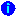Preparation in classical physics). The new phenomena revealed by quantum mechanics will make it necessary to reflect upon the notion of preparation more deeply. To this end, let us consider some further experiments: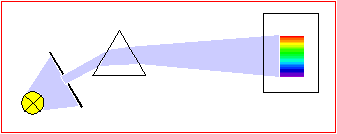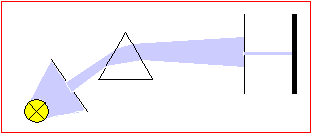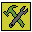Experiment 1: In a darkened room, white light from an incandescent lamp is collimated by a slit and passes through a prism (figure left). On the screen, you see the colored rainbow spectrum known from elementary optics. Select a small range of colors (e. g. green) by placing a second slit behind the prism (figure right). This prepares nearly monochromatic light (light of a single color).

One supposes that light of a specific color possesses a certain property, because it deflected by a well-defined angle a through the prism. This idea can be tested as follows:Experiment 2: The light beam created in the first experiment is passed through a second prism (figure below). Doing this experiment reveals that the light beam is not spread out again by the second prism as the original white light beam was spread into a spectrum by the first prism. As we conjectured, it is deflected by just the same angle a as the selected light in the first prism.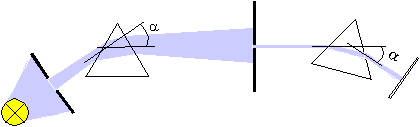The light with a well-defined color that has been created in the first experiment actually possesses the property "is deflected through a certain angle". The original white light from the incandescent lamp did not possess this property; it was spread out by the prism. As we know, the property discovered in this experiment is identical to the property "has a definite wavelength". The property "wavelength" determines the angle of deflection as well as the property "color".

Light with these properties is produced by preparation. In this case, the preparation is carried out by traversing the prism and the second slit. Generally, physical systems with definite properties are brought about by an act of preparation.

#### b) Preparation and Test

What is the purpose of the second prism in experiment 2? It has a different significance than the one that did the preparation. It performs a test measurement to check whether the intended preparation has indeed been performed.

This scheme, comprising the preparation and test stages can be employed in a variety of physical situations. First one tries to prepare a certain property (e. g. wavelength, kinetic energy) on a physical object (e. g. light, electron). To make sure that the object really possesses this property, a second (possibly identical) experiment is performed that checks for this property.

If one can predict with certainty that a test yields a certain property in all cases you are allowed to ascribe this property to the physical object. In this case (and only in this case) the object really posseses this property. We will see later that one really needs to pay attention to this prescription. Later we will look at cases where contradictions arise with observed phenomena if we ascribe properties to quantum objects independent of a preparation or a test.

### 5. Preparation and the quantum mechanical states

After having introduced the notion of preparation we may ask what is being prepared. A seemingly obvious answer in the case of the double-slit experiment might be: "a single electron that leaves the source". However, this answer fails to characterize the specific outcome of a preparation. As already noted above, two electrons that are subject to identical preparation procedures will in general not end at the same position on the screen. A preparation procedure does not determine the result of single events.

This observation led us in Section 2 to consider probabilities. A complete preparation leads to reproducible predictions about the probabilities of events. In the double-slit experiment, the source prepares electrons with definite kinetic energy, thus they possess the property "kinetic energy". This preparation determines the probability distribution on the screen given the separation and the widths of the slits. We have been able to reliably predict the places where many electrons and where few electrons will be detected.

The preparation is independent of the subsequent interaction and measurement. We could for example perform a scattering experiment with the above electrons, and measure the distribution of scattering angles after the scattering. Therefore the preparation has to determine the probabilities for all possible experiments that can be performed on the prepared object. To make this connection between preparation and measured probability distributions more explicit, one introduces the notion of the quantum mechanical state. It is identified with the specification of a probability distribution for each possible measurement.

Mathematically, the quantum mechanical state is described by a wave function. With certain mathematical operations, it is possible to extract from the wave function the probabilities of all conceivable experiments. Quantitative statements then become possible. The conceptual connection between preparation, state, wave function and probability distribution is illustrated in the graphics below.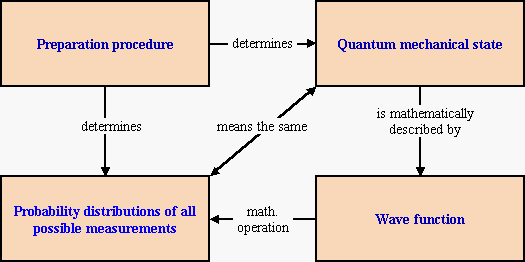In later lessons, we will discuss examples of preparation procedures and their connections to wave functions and probabilities. However, in the next lessons we will aim for a more qualitative understanding of quantum mechanical phenomena.

Do you have comments or questions? Send an e-mail to the discussion group:### Sources:

 L. Eisenbud, The Conceptual Foundations of Quantum Mechanics, Van Nostrand Reinhold, New York 1971.
This book contains a nice introduction to the conceptual issues of quantum mechanics. The notion of dynamical properties is strongly stressed here. Preparation of properties is illustrated with Newton`s prism experiment.

 L. Ballentine, Quantum Mechanics. A Modern Development, World Scientific, Singapore 1998.
Ballentine is one of the most prominent advocates of the ensemble interpretation of quantum mechanics. In his book, therefore, preparation and measurement are clearly distinguished. The quantum mechanical state concept is clearly discussed. Section 5 of the present lesson rests mainly on Ballentine`s Chapter 2.1.

 Kansas State University Physics Education Group (D. Zollman et al.), Visual Quantum Mechanics, Manhattan, Kansas, 1998.
The Visual Quantum Mechanics Project pursued at the Kansas State University provides hands-on materials for introducing quantum mechanics on a qualitative level. The working sheet and Section 2 rest on these materials.

 R. Müller, H. Wiesner, Das Münchener Unterrichtskonzept zur Quantenmechanik, Manuskript, 1999.
The material developed at the University of Munich is an introduction to quantum mechanics that stresses mainly the conceptual foundations. If not mentioned otherwise, the present lesson is based on Chapter 2 of this manuscript.